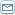# Math or Magic?

 By: Kenneth WilliamsAs someone once said: 'There's an easy way to do something, and an unlimited number of difficult ways'. Take simple division, for example: Suppose you need to divide 43 by 9. The answer is 4 (the first figure of 43) and the remainder is 7 (4 + 3). Similarly 35 divided by 9 is 3 remainder 8. In the Vedic system we use the natural properties of numbers. The number 9 has the property that it is 1 below 10. So every 10 contains one 9 and one remainder. Therefore, in 40, there will be 4 nines and 4 remainder. and so in 43 there must be 4 nines and 7 remainder. This can be developed in many ways. For example to divide 123 by 9 the answer is 13 remainder 6. The first figure of the answer (1 in the 13) is the first figure of 123. The second figure of the answer (3 in the 13) is 1+2 (add the first two figures of 123). The remainder is 6 is 1+2+3 (add all three figures of 123). As B. K. Tirthaji (the man who reconstructed the Vedic system) said: 'its magic until you understand it, and its ematics thereafter'
 Parenting